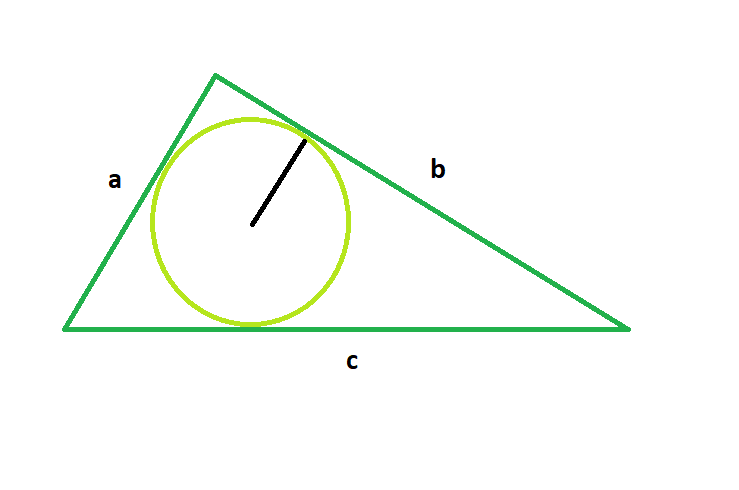# Program to find the Radius of the incircle of the triangle

Given a circle which is the incircle of a triangle whose sides are a, b< and c, the task is to find the radius of this incircle.

Examples:

```Input: a = 2, b = 2, c = 3
Output: 0.566947

Input: a = 3, b = 4, c = 5
Output: 1
```Approach:

Radius of the incircle = area of the triangle / half of perimeter of the triangle
where:
Area of the triangle = √(p*(p-a)*(p-b)*(p-c)
perimeter of the triangle = (a + b + c)

Below is the implementation of the above approach:

## C++

 `// C++ Program to find the radius ` `// of the incircle of the given triangle ` ` `  `#include ` `using` `namespace` `std; ` ` `  `// Function to find the radius ` `// of the incircle ` `float` `findRadiusOfIncircle(``float` `a, ``float` `b, ``float` `c) ` `{ ` ` `  `    ``// the sides cannot be negative ` `    ``if` `(a < 0 || b < 0 || c < 0) ` `        ``return` `-1; ` ` `  `    ``// semi-perimeter of the circle ` `    ``float` `p = (a + b + c) / 2; ` ` `  `    ``// area of the traingle ` `    ``float` `area = ``sqrt``(p * (p - a) * (p - b) * (p - c)); ` ` `  `    ``// Radius of the incircle ` `    ``float` `radius = area / p; ` ` `  `    ``// Return the radius ` `    ``return` `radius; ` `} ` ` `  `// Driver code ` `int` `main() ` `{ ` ` `  `    ``// Get the sides of the traingle ` `    ``float` `a = 2, b = 2, c = 3; ` ` `  `    ``// Find the radius of the incircle ` `    ``cout << findRadiusOfIncircle(a, b, c) ` `         ``<< endl; ` ` `  `    ``return` `0; ` `} `

## Java

 `// Java Program to find the radius ` `// of the incircle of the given triangle ` `import` `java.io.*; ` ` `  `class` `GFG { ` `   `  `// Function to find the radius ` `// of the incircle ` `static` `float` `findRadiusOfIncircle(``float` `a, ``float` `b, ``float` `c) ` `{ ` ` `  `    ``// the sides cannot be negative ` `    ``if` `(a < ``0` `|| b < ``0` `|| c < ``0``) ` `        ``return` `-``1``; ` ` `  `    ``// semi-perimeter of the circle ` `    ``float` `p = (a + b + c) / ``2``; ` ` `  `    ``// area of the traingle ` `    ``float` `area = (``float``)Math.sqrt(p * (p - a) * (p - b) * (p - c)); ` ` `  `    ``// Radius of the incircle ` `    ``float` `radius = area / p; ` ` `  `    ``// Return the radius ` `    ``return` `radius; ` `} ` ` `  `// Driver code ` ` `  `    ``public` `static` `void` `main (String[] args) { ` `         `  `    ``// Get the sides of the traingle ` `    ``float` `a = ``2``, b = ``2``, c = ``3``; ` ` `  `    ``// Find the radius of the incircle ` `    ``System.out.println( findRadiusOfIncircle(a, b, c)); ` `         `  `    ``} ` `} ` ` ``// This code is contributed by ajit `

## Python 3

 `# Python Program to find the radius  ` `# of the incircle of the given triangle  ` ` `  `# from math lib. import everything ` `from` `math ``import` `*` ` `  `# Function to find the radius  ` `# of the incircle  ` `def` `findRadiusOfIncircle(a, b, c) : ` ` `  `    ``# the sides cannot be negative  ` `    ``if` `(a < ``0` `or` `b < ``0` `or` `c < ``0``) : ` `        ``return` `-``1` ` `  `    ``# semi-perimeter of the circle  ` `    ``p ``=` `(a ``+` `b ``+` `c) ``/` `2` ` `  `    ``# area of the traingle  ` `    ``area ``=` `sqrt(p ``*` `(p ``-` `a) ``*`  `               ``(p ``-` `b) ``*` `(p ``-` `c))  ` ` `  `    ``# Radius of the incircle  ` `    ``radius ``=` `area ``/` `p  ` ` `  `    ``# Return the radius  ` `    ``return` `radius  ` ` `  `# Driver code      ` `if` `__name__ ``=``=` `"__main__"` `: ` ` `  `    ``# Get the sides of the traingle  ` `    ``a, b, c ``=` `2``, ``2``, ``3` ` `  `    ``# Find the radius of the incircle  ` `    ``print``(``round``(findRadiusOfIncircle(a, b, c), ``6``))  ` ` `  `# This code is contributed by ANKITRAI1 `

## C#

 `// C# Program to find the radius ` `// of the incircle of the given triangle ` `using` `System; ` ` `  `class` `GFG  ` `{ ` ` `  `// Function to find the radius ` `// of the incircle ` `public` `static` `float` `findRadiusOfIncircle(``float` `a, ` `                                         ``float` `b,  ` `                                         ``float` `c) ` `{ ` ` `  `    ``// the sides cannot be negative ` `    ``if` `(a < 0 || b < 0 || c < 0) ` `        ``return` `-1; ` `     `  `    ``// semi-perimeter of the circle ` `    ``float` `p = (a + b + c) / 2; ` `     `  `    ``// area of the traingle ` `    ``float` `area = (``float``)Math.Sqrt(p * (p - a) *  ` `                          ``(p - b) * (p - c)); ` `     `  `    ``// Radius of the incircle ` `    ``float` `radius = area / p; ` `     `  `    ``// Return the radius ` `    ``return` `(``float``)(radius); ` `} ` ` `  `// Driver code ` `public` `static` `void` `Main() ` `{ ` ` `  `    ``// Get the sides of the traingle ` `    ``float` `a = 2, b = 2, c = 3; ` ` `  `    ``// Find the radius of the incircle ` `    ``Console.WriteLine(findRadiusOfIncircle(a, b, c)); ` `} ` `} ` ` `  `// This code is contributed ` `// by Shivi_Aggarwal `

## PHP

 `

Output:

```0.566947
```

My Personal Notes arrow_drop_upCheck out this Author's contributed articles.

If you like GeeksforGeeks and would like to contribute, you can also write an article using contribute.geeksforgeeks.org or mail your article to contribute@geeksforgeeks.org. See your article appearing on the GeeksforGeeks main page and help other Geeks.

Please Improve this article if you find anything incorrect by clicking on the "Improve Article" button below.